# Essentials of Economics Study Set 12

## Quiz 22 : Money Growth and InflationStudy FlashcardsLooking for Economics Homework Help?

## Quiz 22 :Money Growth and Inflation

Question TypeIn what sense is inflation like a tax? How does thinking about inflation as a tax help explain hyperinflation?
Free
Essay

With the deduction of Tax, we lose money along with the purchasing power. In the same way with inflation, the purchasing power of money decreases. In this sense, the inflation is like a tax.
With the government increasing the money supply in the economy increases rapidly effecting in the more loss of purchasing power resulting in hyperinflation. In these circumstances, the government, for its financial funding, uses the inflation tax instead of tax.

Tags
Choose question tagAccording to the quantity theory of money and the Fisher effect, if the central bank increases the rate of money growth,
Free
Multiple Choice

Effect of money growth:
According to the quantity theory of money and the Fisher effect, increase in rate of money growth causes the rate of inflation and nominal variables to increase, but the real variables remain constant.
Thus, the option 'a' is correct.

Tags
Choose question tagIf the tax rate is 40 percent, compute the before-tax real interest rate and the after-tax real interest rate in each of the following cases. a. The nominal interest rate is 10 percent, and the inflation rate is 5 percent. b. The nominal interest rate is 6 percent, and the inflation rate is 2 percent. c. The nominal interest rate is 4 percent, and the inflation rate is 1 percent.
Free
Essay

Real interest rate:
Nominal interest indicates how fast the deposited amount in the saving account will increase.
Real interest rate indicates how fast the purchasing power of the saving account will increase over time. Increase in rate of inflation causes the purchasing power to fall; hence, the real interest rate can be obtained by using the following formula: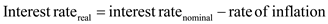…… (1)
The nominal interest rate, after tax is levied, can be calculated as follows: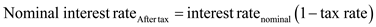…… (2)
a.
Nominal rate of interest is 10 percent and rate of inflation is 5 percent. Substitute the respective values in Equation (1) to obtain the real interest rate.
Real interest rate before tax: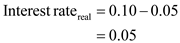Real interest rate after tax: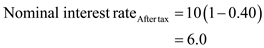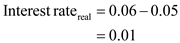b.
Nominal rate of interest is 6 percent and rate of inflation is 2 percent. If the tax rate is 40 percent, then the real rate of interest before tax can be obtained by using the Equation (1) and after tax can be obtained by using the Equation (1) and (2).
Real interest rate before tax: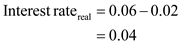Real interest rate after tax: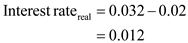c.
Nominal rate of interest is 4 percent and rate of inflation is 1 percent. If the tax rate is 40 percent, then the real rate of interest before tax and after tax can be obtained by using the Equation (1).
Real interest rate before tax: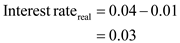Real interest rate after tax: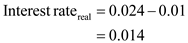Tags
Choose question tagSuppose that a country's inflation rate increases sharply. What happens to the inflation tax on the holders of money? Why is wealth that is held in savings accounts not subject to a change in the inflation tax? Can you think of any way holders of savings accounts are hurt by the increase in the inflation rate?
Essay
Tags
Choose question tagWhat are the costs of inflation? Which of these costs do you think are most important for the U.S. economy?
Essay
Tags
Choose question tagExplain the difference between nominal and real variables and give two examples of each. According to the principle of monetary neutrality, which variables are affected by changes in the quantity of money?
Essay
Tags
Choose question tagLet's consider the effects of inflation in an economy composed of only two people: Bob, a bean farmer, and Rita, a rice farmer. Bob and Rita both always consume equal amounts of rice and beans. In 2013, the price of beans was $1 and the price of rice was$3. a. Suppose that in 2014 the price of beans was $2 and the price of rice was$6. What was inflation? Was Bob better off, worse off, or unaffected by the changes in prices? What about Rita? b. Now suppose that in 2014 the price of beans was $2 and the price of rice was$4. What was inflation? Was Bob better off, worse off, or unaffected by the changes in prices? What about Rita? c. Finally, suppose that in 2014 the price of beans was $2 and the price of rice was$1.50. What was inflation? Was Bob better off, worse off, or unaffected by the changes in prices? What about Rita? d. What matters more to Bob and Rita-the overall inflation rate or the relative price of rice and beans?
Essay
Tags
Choose question tagAccording to the quantity theory of money, which variable in the quantity equation is most stable over long periods of time?
Multiple Choice
Tags
Choose question tagThe classical principle of monetary neutrality states that changes in the money supply do not influence _________ variables and is thought most applicable in the _________ run.
Multiple Choice
Tags
Choose question tagIf nominal GDP is $400, real GDP is$200, and the money supply is $100, then Multiple Choice Answer: Tags Choose question tagRecall that money serves three functions in the economy. What are those functions? How does inflation affect the ability of money to serve each of these functions? Essay Answer: Tags Choose question tagSuppose that this year's money supply is$500 billion, nominal GDP is $10 trillion, and real GDP is$5 trillion. a. What is the price level? What is the velocity of money? b. Suppose that velocity is constant and the economy's output of goods and services rises by 5 percent each year. What will happen to nominal GDP and the price level next year if the Fed keeps the money supply constant? c. What money supply should the Fed set next year if it wants to keep the price level stable? d. What money supply should the Fed set next year if it wants an inflation of 10 percent?
Essay
Tags
Choose question tagIf an economy always has inflation of 10 percent per year, which of the following costs of inflation will it NOT suffer?
Multiple Choice
Tags
Choose question tagSuppose that changes in bank regulations expand the availability of credit cards so that people need to hold less cash. a. How does this event affect the demand for money? b. If the Fed does not respond to this event, what will happen to the price level? c. If the Fed wants to keep the price level stable, what should it do?
Essay
Tags
Choose question tagAccording to the Fisher effect, how does an increase in the inflation rate affect the real interest rate and the nominal interest rate?
Essay
Tags
Choose question tagAccording to the quantity theory of money, what is the effect of an increase in the quantity of money?
Essay
Tags
Choose question tagIt is sometimes suggested that the Fed should try to achieve zero inflation. If we assume that velocity is constant, does this zero-inflation goal require that the rate of money growth equal zero? If yes, explain why. If no, explain what the rate of money growth should equal.
Essay